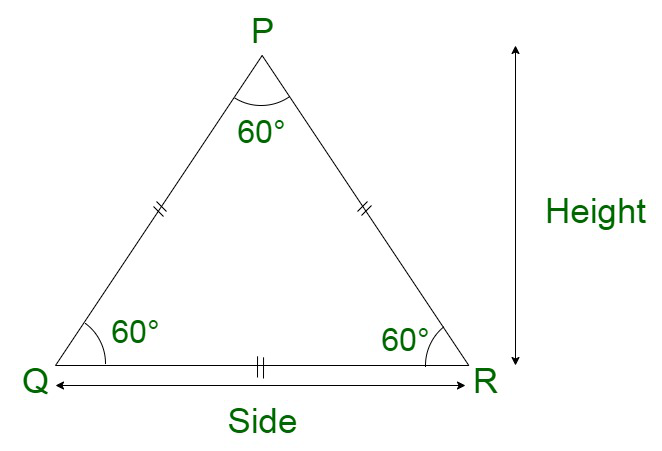# How to find the altitude of an equilateral triangle given the perimeter?

An equilateral triangle is a geometrical 2D figure which has all three sides equal. All the edges subtend an angle of 60° at the corners. Therefore, the sum of all the angles of the triangle is 180°.Properties of an equilateral triangle

• All three sides of an equilateral triangle are equal.
• All the three angles are equal i.e 60° each.
• A regular polygon has three equal sides.

### Perimeter of an Equilateral Triangle

The perimeter of a square is referred to as the total length of the boundary enclosing the geometrical figure.

Perimeter of equilateral triangle = Sum of all sides of an equilateral triangle

Let us assume a to be the side of an equilateral triangle.

Since, we know, all the sides of the equilateral triangle are equal.

Perimeter of equilateral triangle = a + a + a

Perimeter of equilateral triangle = 3a

### Altitude of an equilateral triangle

Perimeter of an equilateral triangle can be calculated using the altitude of the triangle.

Let us assume ‘a’ to be the side of an equilateral triangle.

Since,

Height of an Equilateral Triangle =Now, let us assume P to be the perimeter of the equilateral triangle.

P = side + side + side

Since, all the sides are equal,

P = 3a

Substituting values,

Height =### Sample Questions

Question 1. Calculate the perimeter of an equilateral triangle having a height 20√3 cm.

Solution:

Here we have to find the Perimeter of the equilateral triangle with the height 20√3 cm

Formula for calculating perimeter using height is given below

Height =Here a is the side of the equilateral triangle

20√3 =a =a = 40 cm

Now further finding perimeter of the equilateral triangle

Perimeter of equilateral triangle = side + side + side = 3a

Perimeter of equilateral triangle = 3 × 40

Perimeter of equilateral triangle = 120 cm

Question 2. Assume the height of an Equilateral triangle is 100√3 m, then find its perimeter?

Solution:

Here we have to find the Perimeter of the equilateral triangle with the height 100√3 m

Formula for calculating perimeter using height is given below

Height =Here a is the side of the equilateral triangle

100√3 =a =a = 200 m

Now further finding perimeter of the equilateral triangle

Perimeter of equilateral triangle = side + side + side = 3a

Perimeter of equilateral triangle = 3 × 200

Perimeter of equilateral triangle = 600 m

Question 3. Find the perimeter of an equilateral triangle with each side 22 m. Also, find its height?

Solution:

First find the perimeter of an equilateral triangle

Perimeter of equilateral triangle = side + side + side = 3a

Perimeter of equilateral triangle = 3 × 22

Perimeter of equilateral triangle = 66 m

Further finding the height of the equilateral triangle

Height =Here a is side of the equilateral triangle

Height =Height of the equilateral triangle = 11√3

Question 4. If the perimeter of an equilateral triangle is 99 cm, then find the height of the equilateral triangle?

Solution:

First we have to find the side of equilateral triangle

As we know that

Perimeter of equilateral triangle = side + side + side = 3a

As given in the question

Perimeter of equilateral triangle = 99 cm

3a = 99 cm

a =a = 33 cm

Hence the side of triangle is 33 cm

Further finding the height of the equilateral triangle

Height =Here a is side of the equilateral triangle

Height =Height of the equilateral triangle = 16.5√3

Question 5. Find out the perimeter of an equilateral triangle having a height of 200 cm.

Solution:

Here we have to find the Perimeter of the equilateral triangle with the height 200 cm

Formula for calculating perimeter using height is given below

Height =Here a is the side of the equilateral triangle

200 =a =a = 230.94 cm

Now further finding perimeter of the equilateral triangle

Perimeter of equilateral triangle = side + side + side = 3a

Perimeter of equilateral triangle = 3 × 230.94

Perimeter of equilateral triangle = 692.82 cm

Whether you're preparing for your first job interview or aiming to upskill in this ever-evolving tech landscape, GeeksforGeeks Courses are your key to success. We provide top-quality content at affordable prices, all geared towards accelerating your growth in a time-bound manner. Join the millions we've already empowered, and we're here to do the same for you. Don't miss out - check it out now!

Related Tutorials